# The magic kernel

## What is the magic kernel?

The “magic kernel” is a method of resampling images that gives amazingly clear results (free of “aliasing” artifacts, free of “ringing”, and free of “width beat” for thin features). The original and simplest version, that doubles or halves the size of an image, is lightning fast (requiring only a few integer additions and bit-shifts).

I first came across the simple image-doubling magic kernel in 2006 in the source code for a popularly-used JPEG library. Between 2006 and 2012 I investigated its remarkable properties in depth, and generalized it to allow resizing an image to any size.

Adding a simple three-tap sharpening step to the output of the generalized magic kernel yields an effective kernel that is a close approximation of the ideal sinc resampling kernel, which possesses Fourier space properties that suppress aliasing artifacts at low frequencies (folded over from even multiples of the Nyquist frequency) better than Lanczos kernels, due to higher-order zeroes at those critical frequencies. This factorization also allows an effective implementation of the resulting “Magic Kernel Sharp” algorithm using hardware-assisted libraries that only provide filtering and un-antialiased downsampling routines.

For cases in which extra “pop” is desired in the final downsampled image, the coefficient of the sharpening step can be simply increased beyond that needed for the ideal response, yielding a “Magic Kernel Sharp+” algorithm with the same computational performance as the original.

Magic Kernel Sharp has been used for server-side resizing of images on Facebook since early 2013, and Magic Kernel Sharp+ has been used for server-side resizing of images on Instagram since 2015.

## Where did the magic kernel come from?

In 2006 I was seeking to minimize the “blocking artifacts” caused by heavy use of JPEG compression (see here). In doing so, I made use of a popularly-used JPEG library, written in C, created by the Independent JPEG Group (IJG). Standard JPEG compression stores the two channels of chrominance data at only half-resolution, i.e., only one Cr and one Cb value for every 2 x 2 block of pixels. In reconstructing the image, it is therefore necessary to “upsample” the chrominance channels back to full resolution.

The simplest upsampling method is to simply replicate the values in each 2 x 2 block of pixels. But the IJG library also contained an option for “fancy upsampling” of the chrominance. The following example (twice real size) shows the difference in the resulting reconstructions (note, particularly, the edges between red and black):“Replicated” upsampling“Fancy” upsampling

Intrigued, I examined the C source code for this “fancy” upsampling. To my amazement, I discovered that it was nothing more than a kernel of {1/4, 3/4, 3/4, 1/4} in each direction (where, in this notation, the standard “replication” kernel is just {0, 1, 1, 0}).

I decided to try this kernel out on all channels of a general uncompressed image, which has the effect of doubling the size of the image in each direction. To my astonishment, I found that it gave astoundingly good results for repeated upsamplings, no matter how many times it was applied. I therefore dubbed it the “magic kernel”. Even more amazingly, the results were, visually, far superior to the bicubic resampling in common use at that time in Photoshop and GIMP.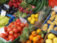Original imageMagic kernel onceMagic kernel twiceMagic kernel thriceBicubic: note the the artifacts

Likewise, applying it as a downsampling kernel yields beautifully antialiased images:New original imageMagic kernel onceMagic kernel twiceMagic kernel thrice

(Note, however, that these images are slightly more blurred than they need to be; this is related to the required post-sharpening step mentioned above, which will be discussed shortly.)

Flabbergasted, and unable to find this kernel either in textbooks or on the web, I asked on the newsgroups whether it was well-known (see here and here). It appeared that it was not.

## Why is the magic kernel so good?

The “correct” antialiasing kernel is a sinc function. In practice, the infinite support and negative values in the sinc function necessitate approximations (such as the Lanczos kernels).

The question, then, was obvious: how on Earth could the simple kernel {1/4, 3/4, 3/4, 1/4} out-perform such “correct” kernels when enlarging an image?

The answer to that question seems to have two parts.

Firstly, as can be seen from the newsgroup discussions following my above postings, the magic kernel is effectively a very simple approximation to the sinc function, albeit with the twist that the new sampling positions are offset from the original positions. (This is obvious when one considers the “tiling” of the original JPEG chrominance implementation, but is not an obvious choice from the point of view of sampling theory.) Charles Bloom noted in 2011 that the magic kernel can be factorized to a nearest-neighbor upsampling to the “doubled” (or “tiled”) grid, followed by convolution with a regular {1/4, 1/2, 1/4} kernel in that new space.

Secondly, it turns out that an arbitrary number of repeated applications of this kernel results in a sequence of kernels with unique and extremely desirable properties. This is the topic of the next section.

## The continuum magic kernel

In investigating why the magic kernel performed miraculously well when applied repeatedly to images, I discovered empirically that, when convolved with itself an arbitrary number of times, the resulting kernel was always of a “tri-parabolic” form, which allows the kernel to “fit into itself” when convolved with a constant source signal.

Taking this to its logical conclusion, I looked at what would happen if a signal were upsampled with the magic kernel an infinite number of times. If we denote by x the coordinate in the original sampled space (where the original signal is sampled at integral values of x), then I found that the infinitely-upsampled continuum magic kernel is given analytically byHere is a graph of the function: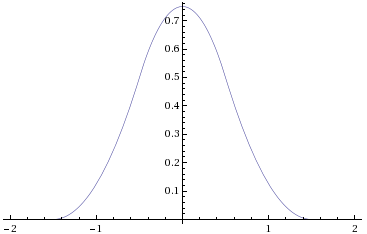This doesn’t look much like a sinc function at all. So how and why does it work so well for enlarging an image?

Firstly, m(x) has finite support: a given sample of the original signal only contributes to the region from 1.5 spacings to the left to 1.5 spacings to the right of its position. This is extremely good in terms of practical implementation: other kernels have wider support.

Secondly, m(x) is non-negative. This avoids introducing “ringing”, and ensures that the output image has exactly the same range as the input image.

Thirdly, m(x) is continuous and has continuous first derivative everywhere, including at the ends of its support. This property ensures that convolving with the magic kernel yields results that “look good”: discontinuities in the kernel or its first derivative generally lead to artifacts that can be detected by the human eye.

Fourthly, the shape of m(x) visually resembles that of a gaussian, which (if one didn’t know anything about Fourier theory) would be the mathematician’s natural choice for an interpolation kernel. The standard deviation of m(x) can be calculated from the above functional form: it is 1/2. Comparing m(x) with a Gaussian with the same standard deviation shows that the resemblance is not just an illusion: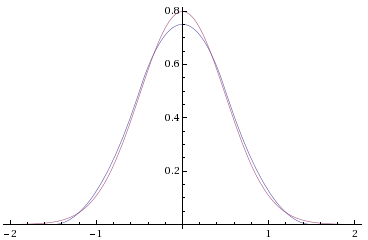Remarkably, the seminal work in pyramid encoding in the early 1980s found exactly the same Gaussian-like properties for a five-tap filter with coefficients {0.05, 0.25, 0.4, 0.25, 0.05} (see here and here).

In contrast, a sinc function has oscillatory lobes that are not an intuitive choice for a smooth interpolation function, and require forced truncation in practice.

Unlike a gaussian, the finite support of m(x) means that only three adjacent copies of it are necessary for it to “fit into itself”. We can therefore see why a truncation of a gaussian to finite support (needed for practical purposes) leads to artifacts.

## Magic Kernel Sharp

Consider the following thought experiment: what if we applied the continuum magic kernel to an image, but with no resizing at all? I.e., what if we simply applied it as a filter, with the output sampling grid being identical to the input sampling grid?

It is clear from the above graph that this would result in a slight blurring of the image: from the formula, the weights of the filter, at positions −1, 0, and +1, would be simply {1/8, 3/4, 1/8}.

Now consider applying the sharpening post-filter {−1/4, +3/2, −1/4} to the resulting slightly-blurred image. The convolution yields an overall kernel of {−1/32, 0, +17/16, 0, −1/32}. This is not a delta function, but is very close to it: there are now zeroes at positions ±1, with −3% lobes at positions ±2, and a corresponding +6% excess at position 0. Rather than the blurring of the original continuum magic kernel, we now have a very slight residual sharpening of the image.

Let us now make the following ansatz: to resize an image, we should apply the Magic Kernel to determine resampling weights, and only after resizing we should apply the sharpening filter {−1/4, +3/2, −1/4} to the results.

It is immediately clear that this leads to the overall filter {−1/32, 0, +17/16, 0, −1/32} above in the limit of an enlargment or reduction factor that approaches unity.

Let us look at what it does for factors that differ from unity. Let us denote by k the ratio of each dimension of the output image (in pixels) to that of the input image, so that k < 1 implies a reduction and k > 1 implies an enlargement. For simplicity, we consider just one dimension of the image; since the kernels are separable, we simply need to repeat the resizing for each dimension.

For a reduction, we are mapping many input pixels to one output pixel. Each output pixel location, say, n (integral), corresponds to the input pixel location y = n / k. (E.g. if we were reducing a 200-pixel image to 20 pixels, then k = 1/10. Output pixel position 0 corresponds to input pixel position 0; output pixel position 1 corresponds to input pixel position 10; and so on.) For each such output pixel n, we overlay the continuum magic kernel m(k y − n) above. (E.g. for n = 5 in our example, the middle output pixel, the kernel is maximal for y = n / k = 50, corresponding to x = 0 in the function; it is zero for y < (n − 3/2) / k = 35, corresponding to x < −3/2 in the function; and it is zero for y > (n + 3/2) / k = 65, corresponding to x > +3/2 in the function.) At each integral position in y-space for which the kernel is nonzero, the unnormalized weight is set to the value of the kernel, i.e. m(k y − n). (E.g. at y = 40 it is set to m(40 / 10 − 5) = m(−1) = 1/8.) We then sum all the unnormalized weights, and normalize them so that the resulting set sums to unity. This set of weights is then used to map the input pixels within the support of the kernel to the given output pixel, when summed. (Note that, for a two-dimensional image, the set of weights for each output pixel in each dimension need only be computed once, and reused for all of the rows or columns in that stage of the resizing.)

After this downsizing with the Magic Kernel has been completed, the result is then convolved with the simple three-tap sharpening post-filter {−1/4, +3/2, −1/4} to produce the final Magic Kernel Sharp downsized image.

In the case of an enlargement, a slightly different process is followed. The Magic Kernel m(x) is in this case applied directly in the input image space, i.e. without any scaling. Each output pixel position n (integral) maps to an input pixel location x = n / k. We simply look up the function m(x) for the unnormalized weight to be applied between each integral position x within the support. After summing these weighted contributions, we again apply the simple three-tap sharpening post-filter {−1/4, +3/2, −1/4} to produce the final Magic Kernel Sharp upsized image.

Note that, for any moderately large upsizing factor k, the final sharpening step will have negligible effect on the results, whereas it always has a significant effect in any downsizing process. This is because of the asymmetry in spaces in which the two filters are applied: for downsizing, the Magic Kernel and Sharp filters are both effectively applied in the output space, so the Sharp filter corresponds to subtracting two reduced copies of the Magic Kernel from a multiple of itself, offset one pixel either way; whereas for upsizing, the Sharp filter is applied in a space that has more pixels than the original image, and for large enlargements corresponds to a negligible sharpening of what is a very smooth enlargment of the original image.

## Properties of the Magic Kernel Sharp downsizing algorithm

To further understand how the Magic Kernel Sharp algorithm works for the practically important case of downsizing, let us look at the effective combined kernel of the Magic Kernel,and the Sharp filter, yielding the Magic Kernel Sharp downsampling filter: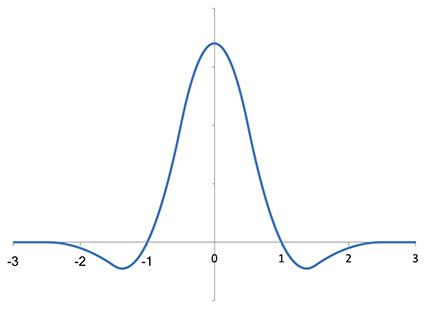Compare this to the Lanczos-2 filter:and the Fourier-ideal sinc function: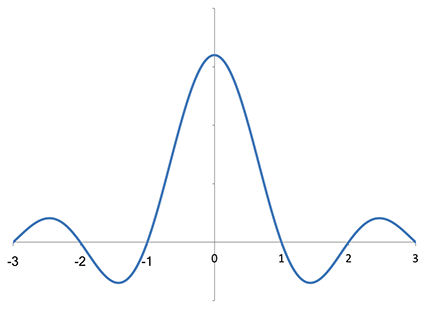(which continues on outside this range, with infinite support). As noted above, the Magic Kernel Sharp filter has zeroes at sampling positions ±1, as with the other filters, but doesn't have zeroes at positions ±2. On the other hand, it has more overall weight in its negative lobes than Lanczos-2. Overall, however, Magic Kernel Sharp and Lanczos-2 are very similar in structure.

To fully appreciate the antialiasing properties of the Magic Kernel Sharp filter, however, we need to look in Fourier space. For reference, let us perform a numerical Fourier transform on the ideal sinc filter, with an arbitrarily-selected number of Fourier coefficients:where we can see the Gibbs phenomenon (ringing) due to the finite number of coefficients chosen. The ideal sinc filter is unity inside the Nyquist domain or Brillouin zone (the Nyquist frequency is 1/2 in the units employed in this graph), and zero outside.

Analyzing the Lanczos-2 filter in the same way, we obtain: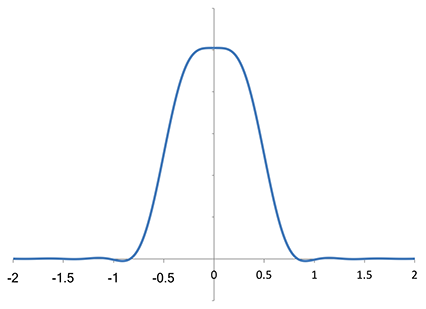We can see that it starts to attenuate high-frequency components within the Nyquist domain; it continues to suppress frequencies outside that domain, and continues to drop until it crosses the axis. Note that it has a zero at twice the Nyquist frequency. This zero is important, because “foldover” will map this frequency to zero frequency under the resampling (i.e. sampling places copies of the original spectrum at every integer, on this scale, so a zero at ±1 means that nothing will end up at DC from the adjacent copies under the sampling).

Analyzing now the Magic Kernel Sharp filter, we obtain: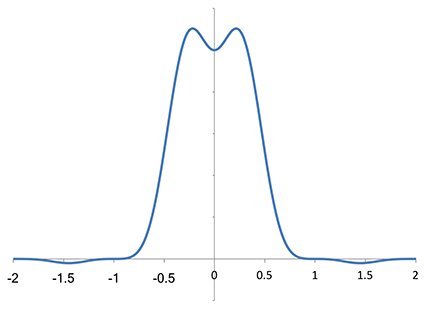It rises slightly before dropping toward zero, but otherwise has a fairly similar shape to the Lanczos-2 spectrum within the first Nyquist zone. But it appears that Magic Kernel Sharp has a higher-order zero at ±1 (and ±2 as well). Let us zoom the vertical axis, to take a closer look: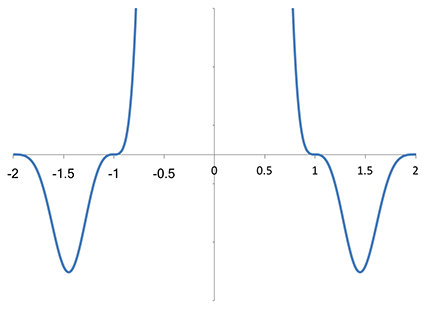It is evident that Magic Kernel Sharp does indeed have a high-order zero at integer values of the sampling frequency. This explains why it has such good visual antialiasing properties: artifacts at low frequencies are particularly visually disturbing; these high-order zeroes ensure that a substantial region of low-frequency space is largely clear of such artifacts.

If we zoom the spectrum of the Lanczos-2 filter to the same scale,we can make a rather startling observation: the Lanczos-2 filter does not actually have a zero at integral multiples of the sampling frequency. Not only is the first zero slightly higher than the sampling frequency, but, more importantly, it is a first-order zero only. Although this zero does a fairly good job of suppressing foldover into the low-frequency domain overall, it is not comparable to the higher-order zero of the Magic Kernel Sharp filter.

## Magic Kernel Sharp downsizing with hardware-assisted library limitations

It may arise in practice that one has hardware-assisted filtering and naive downsampling (e.g. nearest neighbor or bilinear) libraries, but no way of adapting such libraries to perform the full Magic Kernel Sharp downsampling as described above. A satisfactory workaround is as follows: scale up the Magic Kernel by the factor 1 / k as described above, but apply it as a regular filter to the input image. This blurs the original image in its original space, but will only look slightly blurred when downsampled to the final output space. Now apply the best downsampling filter available in the hardware-assisted library (e.g. bilinear, if it is available). This downsampling is not properly antialiased for general images, but it suffices for the image that has been pre-blurred by the Magic Kernel. Now apply the three-tap Sharp filter to the downsampled results. The results are for most practical purposes indistinguishable from those obtained from the full Magic Kernel Sharp algorithm, and are substantially better than using a hardware-assisted downsampling algorithm that is not properly antialiased.

## Magic Kernel Sharp+

In the case that one wishes the output image to have more “pop” (particular after downsizing), one can simply adjust the final three-tap Sharp filter of Magic Kernel Sharp to perform extra sharpening beyond that described above. Namely, instead of {−1/4, +3/2, −1/4}, the weights can be set at {−1/4 − c, +3/2 + 2c, −1/4 − c}, where c is an arbitrary input argument that determines the amount of extra sharpening.

## Source code

In 2011 I wrote a simple implementation of the magic kernel (the simple image-halving and image-doubling versions, as well as the continuum version) in C#. This does not include the sharpening step of Magic Kernel Sharp or Magic Kernel Sharp+, which would have to be added per the description above.